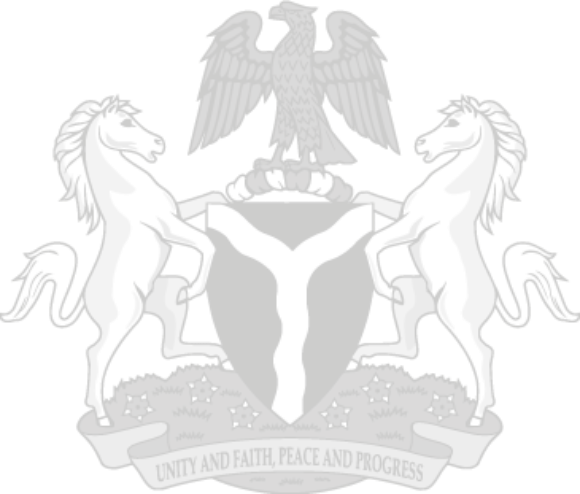## Key Highlights

Revisions for 2020 and 2021 were based on: i. A review of the methodology of compilation. ii. Updated data from NBS data sources, and iii. The exchange rate; the use of investors and exporters window fir international transactions.

The Balance of Trade improved in the Fourth Quarter though it remains negative: Total trade in Q4, 2021 was 11.79% higher when compared to the value recorded in Q3, 2021 and rose by 74.71% compared to the value recorded in the corresponding quarter of 2020. Annual comparison shows that total trade was 57.60% higher in 2021 than the value recorded in 2020.

IMPORTS
The value of total imports in Q4, 2021 increased by 11.33% compared to the value recorded in Q3, 2021 and by 69.41% against the same quarter in 2020 while total imports was 64.11% higher in 2021 than the value recorded in 2020.
• The value of agricultural imports grew by 38.35% in Q4, 2021 compared to the value recorded in Q3, 2021 and it also increased by 118.51% than the value recorded in the corresponding quarter of 2020. Annual growth shows that the value of agricultural imports was 71.76% higher in 2021 than the value recorded in 2020.
• The value of raw material imports grew marginally by 3.80% in Q4, 2021 when compared to the value in Q3, 2021 but was 35.03% higher compared to the value in Q4, 2020. The annual comparison reveals that the value of raw material imports was 37.90% higher than the value recorded in 2020.
• The value of Solid minerals imports was 0.42% lower in Q4, 2021 than the value in Q3, 2021 but increased by 69.73% than the value recorded in Q4, 2020 while the value of solid mineral imports was 54.12% higher in 2021 than the value recorded in 2020.
• The value of Energy goods imports was 507.38% in Q4, 2021 higher than in Q3, 2021 and 124.10% higher than the value recorded in Q4, 2020 while the value of energy goods imports was 86.19% higher in 2021 than the value recorded in 2020.

EXPORTS
The value of total exports in Q4, 2021 increased by 12.27% when compared to the value recorded in Q3, 2021; it also rose by 80.52% compared to the value Q4, 2020. Annual values of total export were 50.99% higher in 2021 than recorded in 2020.
• The value of agricultural goods exports in Q4. 2021 grew by 67.12% compared to Q3 2021 and by137.93% compared to Q4 2020. Annual comparison shows that the value of agricultural exports was 57.02% higher in 2021 than the value recorded in 2020. • The value of raw material goods exports in Q4, 2021 was 65.06% higher than the value the recorded in Q3, 2021. The value recorded in Q4 2021 increased by 429.85% when compared to its value in Q4 2020. In addition, the value of raw material goods exports was 325.54% higher in 2021 than the value recorded in 2020.
• The value of Solid minerals exports in Q4 2021 decreased by 25.95% compared to the value recorded in Q3, 2021 but increased by 201.41% against the corresponding quarter in 2020. The annual comparison of the value of solid mineral exports in 2021 was 320.27% higher than the value recorded in 2020.
• The value of exports of Energy goods in Q4, 2021 rose by 7.17% compared to its value in Q3 2021. This also increased by 308.04% compared to the value recorded in Q4, 2020. In 2021, the value of energy goods exports was 376.52% higher than the value recorded in 2020

Data source: National Bureau of Statistics

### INFOGRAPHICS### Portal & Apps